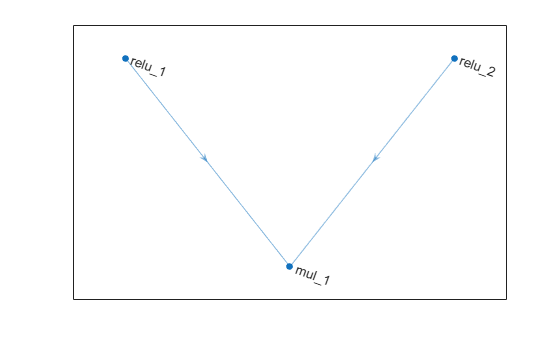multiplicationLayer

Multiplication layer

Description

A multiplication layer multiplies inputs from multiple neural network layers element-wise.

Specify the number of inputs to the layer when you create it. The inputs to the layer have the names 'in1','in2',...,'inN', where N is the number of inputs. Use the input names when connecting or disconnecting the layer by using connectLayers or disconnectLayers, respectively. The size of the inputs to the multiplication layer must be either same across all dimensions or same across at least one dimension with other dimensions as singleton dimensions.

Creation

Description

example

layer = multiplicationLayer(numInputs) creates a multiplication layer that multiplies numInputs inputs element-wise. This function also sets the NumInputs property.

example

layer = multiplicationLayer(numInputs,'Name',name) also sets the Name property.

Properties

expand all

Number of inputs to the layer, specified as a positive integer greater than or equal to 2.

The inputs have the names 'in1','in2',...,'inN', where N is NumInputs. For example, if NumInputs is 3, then the inputs have the names 'in1','in2', and 'in3'. Use the input names when connecting or disconnecting the layer using the connectLayers or disconnectLayers functions.

Layer name, specified as a character vector or a string scalar. For Layer array input, the trainNetwork, assembleNetwork, layerGraph, and dlnetwork functions automatically assign names to layers with Name set to ''.

Data Types: char | string

Input names, specified as {'in1','in2',...,'inN'}, where N is the number of inputs of the layer.

Data Types: cell

Number of outputs of the layer. This layer has a single output only.

Data Types: double

Output names of the layer. This layer has a single output only.

Data Types: cell

Examples

collapse all

Create a multiplication layer with two inputs and the name 'mul_1'.

mul = multiplicationLayer(2,'Name','mul_1')
mul =
MultiplicationLayer with properties:

Name: 'mul_1'
NumInputs: 2
InputNames: {'in1'  'in2'}

Learnable Parameters
No properties.

State Parameters
No properties.

Show all properties

Create two ReLU layers and connect them to the multiplication layer. The multiplication layer multiplies the outputs from the ReLU layers.

relu_1 = reluLayer('Name','relu_1');
relu_2 = reluLayer('Name','relu_2');

lgraph = layerGraph();

lgraph = connectLayers(lgraph,'relu_1','mul_1/in1');
lgraph = connectLayers(lgraph,'relu_2','mul_1/in2');

plot(lgraph);